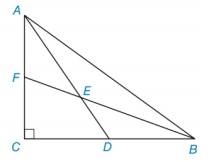Chapter 2.4, Problem 50E### Elementary Geometry for College St...

6th Edition
Daniel C. Alexander + 1 other
ISBN: 9781285195698

#### Solutions

Chapter
Section### Elementary Geometry for College St...

6th Edition
Daniel C. Alexander + 1 other
ISBN: 9781285195698
Textbook Problem
1 views

# Given: In rt Δ A B C , A D ¯ bisects ∠ C A B and B F ¯ bisects ∠ A B C . Find: m ∠ F E DTo determine

The measure of angle mFED.

Explanation

Given:

In right ΔABC, AD¯ bisects CAB and BF¯ bisects ABC.

Definition:

Angle bisector bisects the angle in half.

The sum of the measures of interior angles of a triangle is 180°.

If two lines intersect each other than the vertical angle formed are congruent to each other and their measures are same.

Calculation:

Consider the given figure.

Figure (1)

Consider the right triangle ΔABC,

mABC+mCAB+mC=180°(1)

Substitute 90° for mC in the equation (1).

mABC+mCAB+90°=180°mABC+mCAB=180°90°mABC+mCAB=90°(2)

Since, the angle bisector bisects the angle in half, then from the figure (1)

mBAE=12(

### Still sussing out bartleby?

Check out a sample textbook solution.

See a sample solution

#### The Solution to Your Study Problems

Bartleby provides explanations to thousands of textbook problems written by our experts, many with advanced degrees!

Get Started

#### Find more solutions based on key concepts